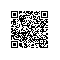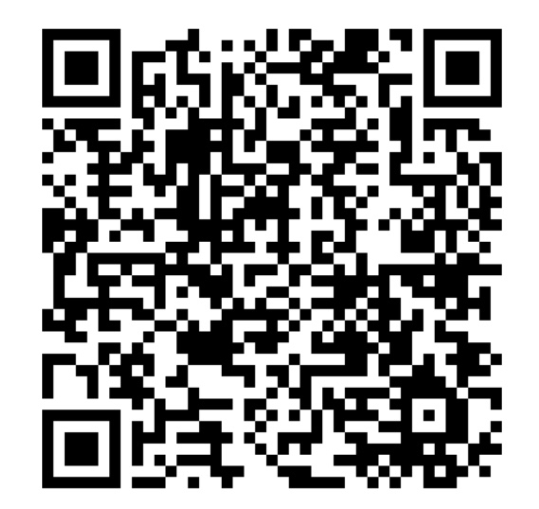# Math.Round()——面试题小结

看下面的例子：
Math.Round(3.44, 1); //Returns 3.4.
Math.Round(3.45, 1); //Returns 3.4.
Math.Round(3.46, 1); //Returns 3.5.

Math.Round(3.445, 1); //Returns 3.4.
Math.Round(3.455, 1); //Returns 3.5.
Math.Round(3.465, 1); //Returns 3.5.

Math.Round(3.450, 1); //Returns 3.4.(补0是无效的)

Math.Round(3.4452, 2); //Returns 3.45.
Math.Round(3.4552, 2); //Returns 3.46.
Math.Round(3.4652, 2); //Returns 3.47.

——（例子来自博客园）使用钉钉扫一扫加入圈子
+ 订阅